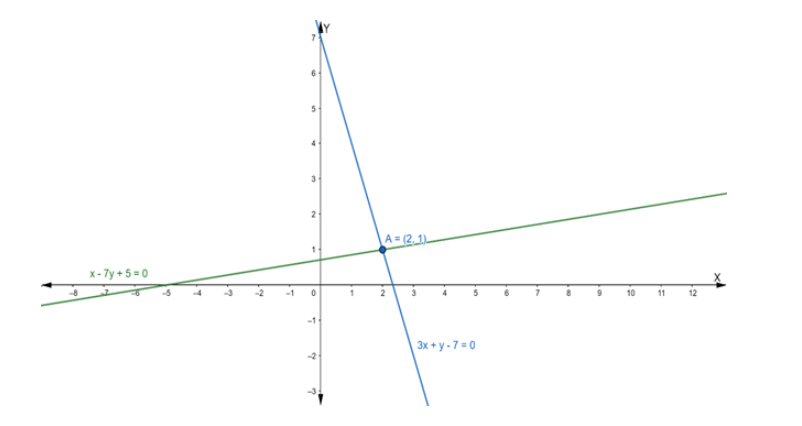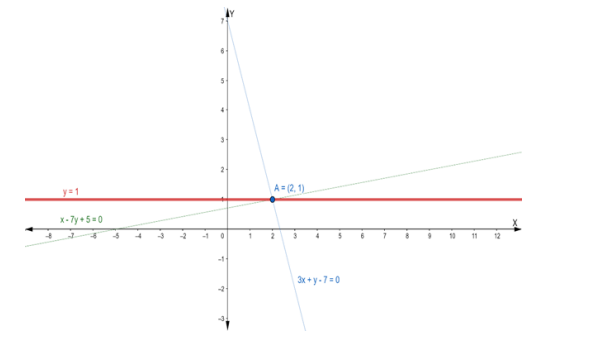# Find the equation of the line through the intersection of the lines`
Question:

Find the equation of the line through the intersection of the lines $x-7 y+5=$ 0 and $3 x+y-7=0$ and which is parallel to $x$-axis.

Solution:

Suppose the given two lines intersect at a point $P\left(x_{1}, y_{1}\right)$. Then, $\left(x_{1}, y_{1}\right)$ satisfies each of the given equations.

$x-7 y+5=0 \ldots$ (i)

$3 x+y-7=0 \ldots$ (ii)

Now, we find the point of intersection of eq. (i) and (ii)

Multiply the eq. (i) by 3, we get

$3 x-21 y+15=0$ ..........(iii)

On subtracting eq. (iii) from (ii), we get

$3 x+y-7-3 x+21 y-15=0$

$\Rightarrow 22 y-22=0$

$\Rightarrow 22 y=22$

$\Rightarrow y=1$

Putting the value of y in eq. (i), we get

$x-7(1)+5=0$

$\Rightarrow x-7+5=0$

$\Rightarrow x-2=0$

$\Rightarrow x=2$

Hence, the point of intersection $\mathrm{P}\left(\mathrm{x}_{1}, \mathrm{y}_{1}\right)$ is $(2,1)$The equation of line parallel to $x$-axis is of the form

y = b where b is some constant

Given that this equation of the line passing through the point of intersection (2, 1)

Hence, point (2, 1) will satisfy the equation of a line.

Putting y = 1 in the equation y = b, we get

y = b

$\Rightarrow 1=b$

or b = 1

Now, the required equation of a line is y = 1